# RD Sharma Solutions for Class 8 Maths Chapter 8 Division of Algebraic Expressions Exercise 8.4

Division of a polynomial by a binomial by using long division method is the concept explained in this Exercise 8.4 of Chapter 8. Solutions are derived in the step by step format as the concept is based on long division method. Our expert tutors have developed the solutions in order to help students focus on their weaker areas. By regular practice, students can solve the problems at ease without any confusion. Students can easily download the RD Sharma Solutions for Class 8 Maths Exercise 8.4 from the links provided below.

## Download the pdf of RD Sharma Solutions for Class 8 Maths Exercise 8.4 Chapter 8 Division of Algebraic Expressions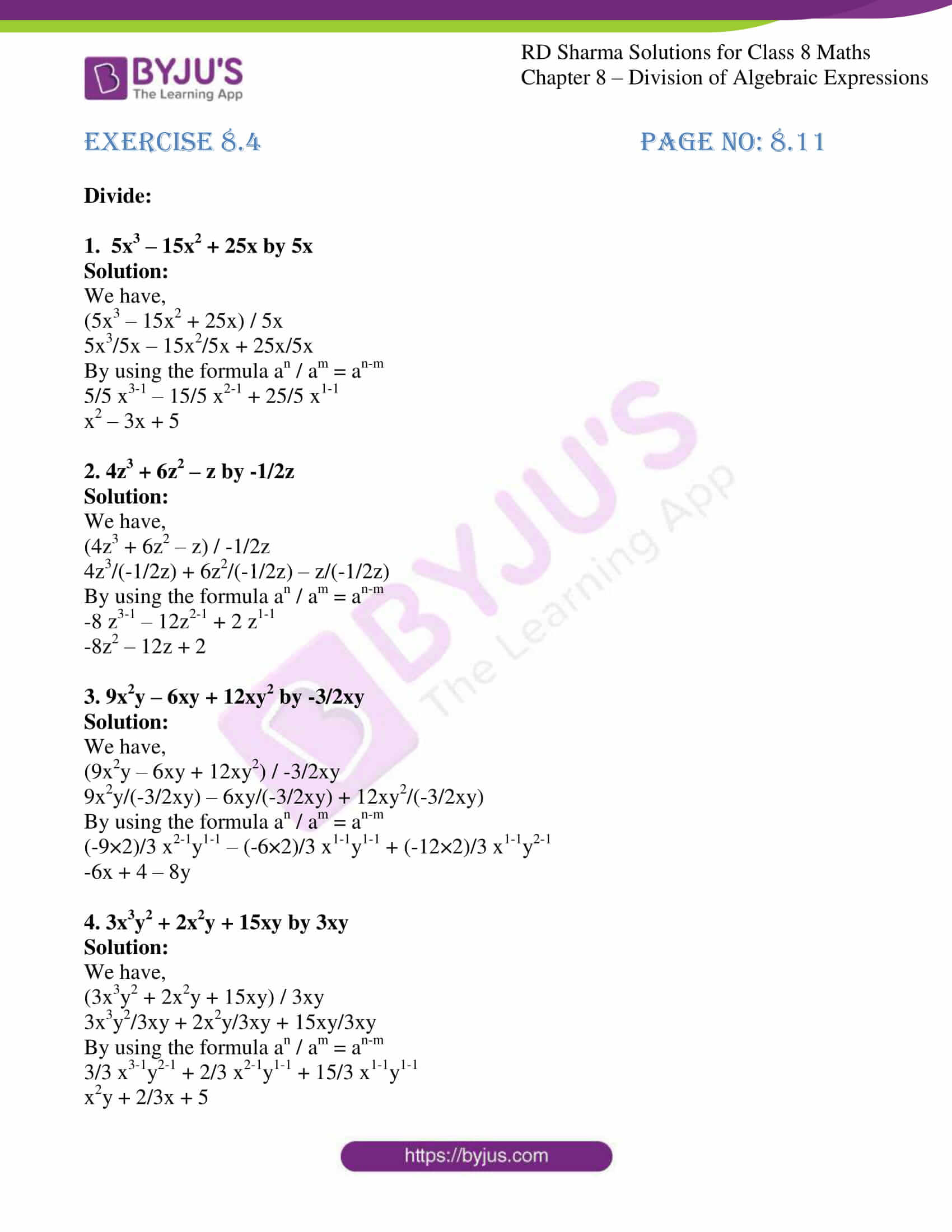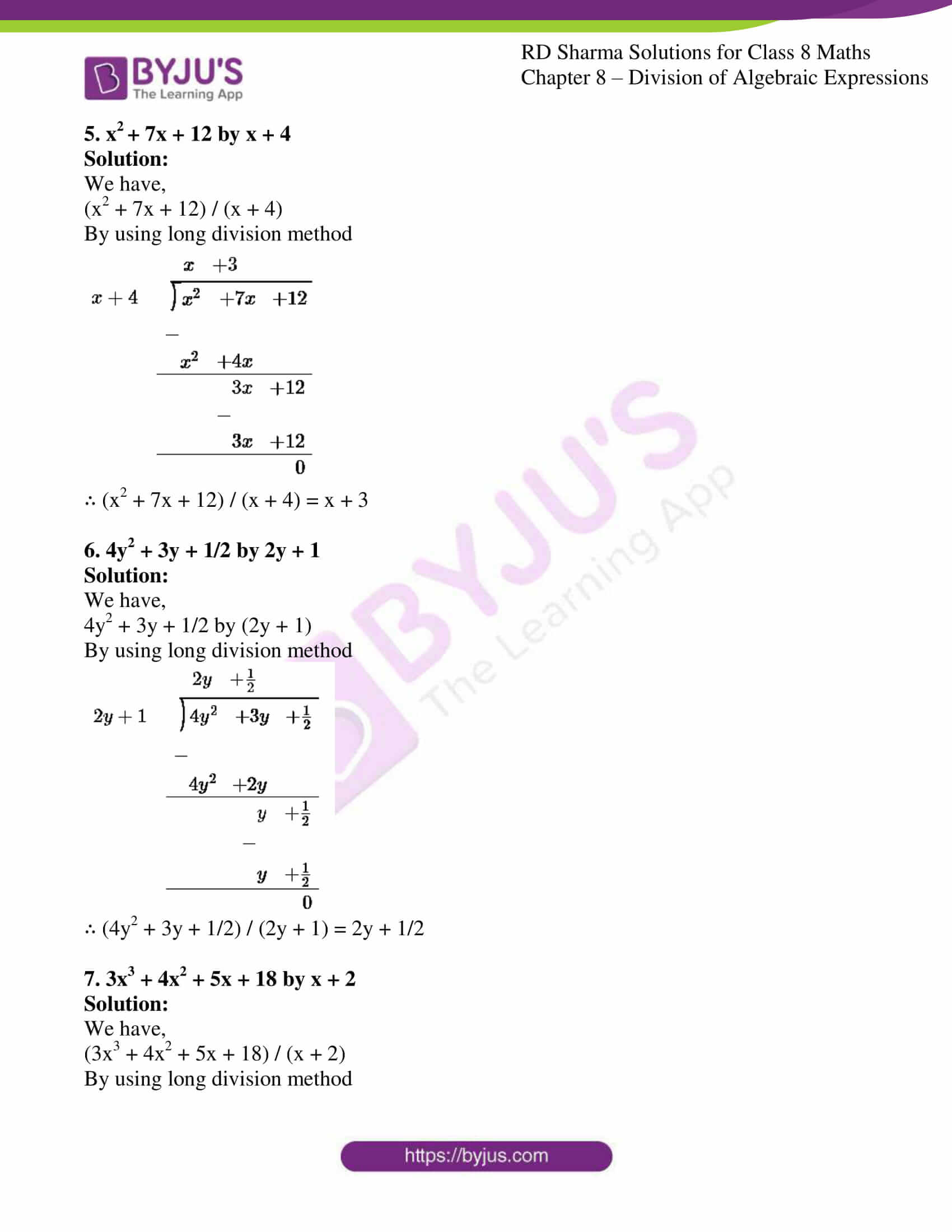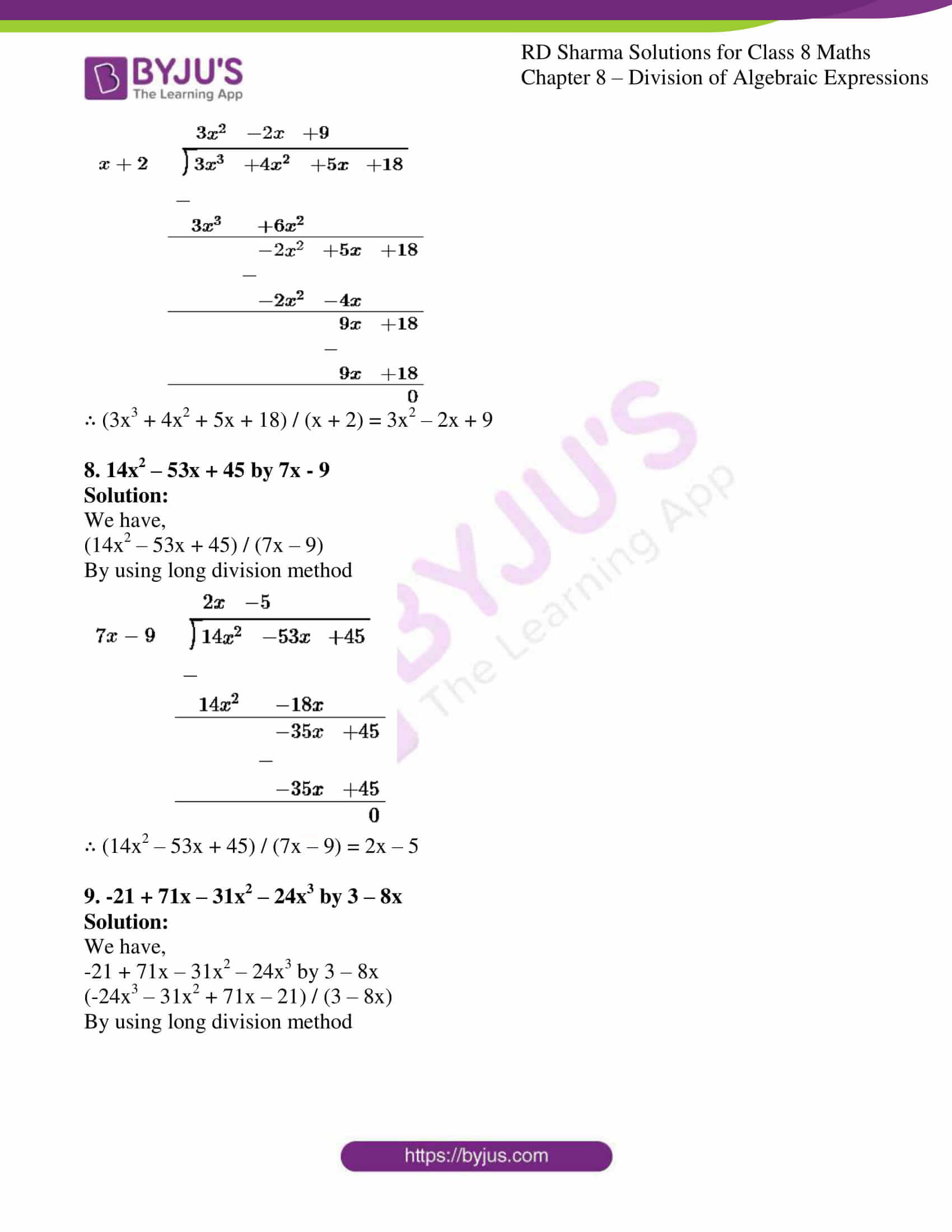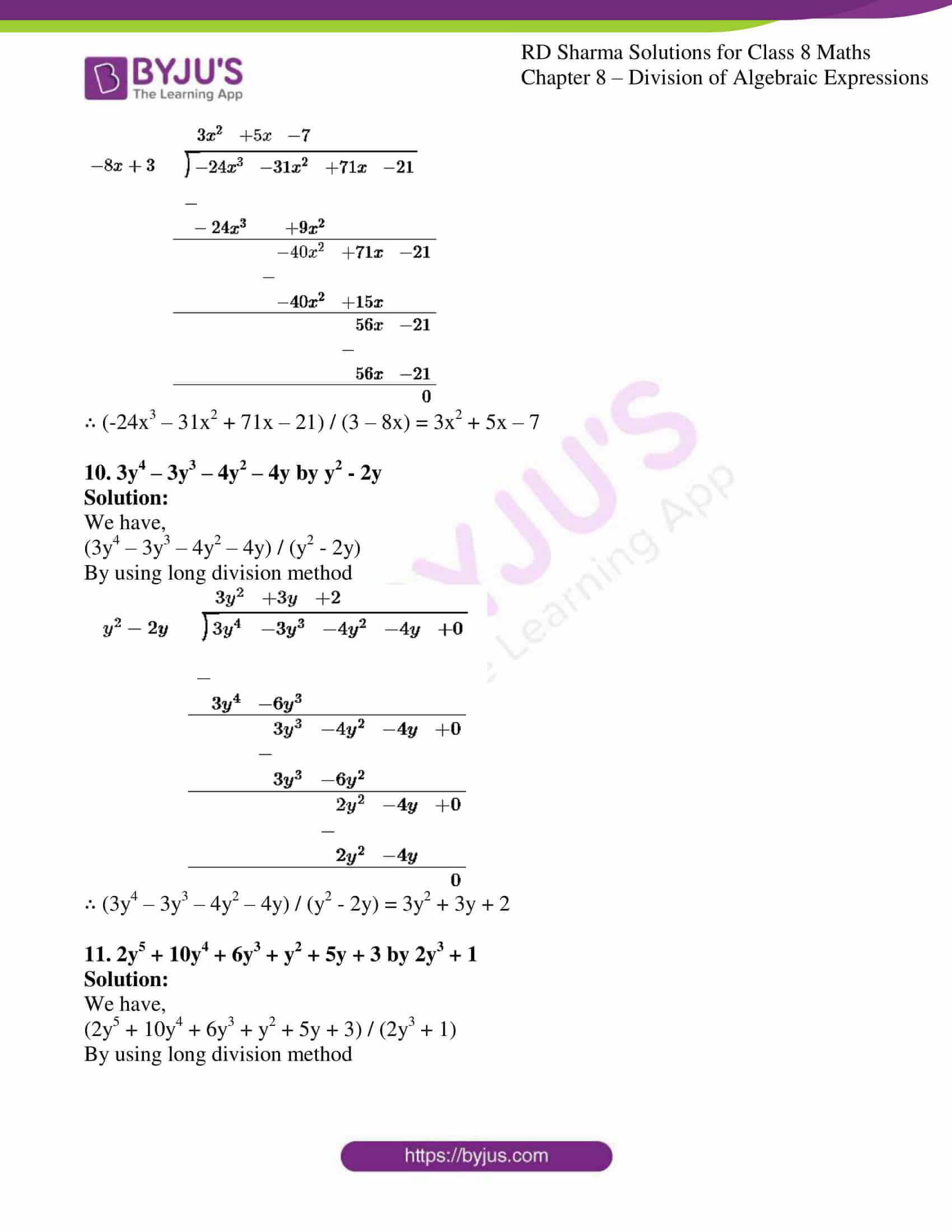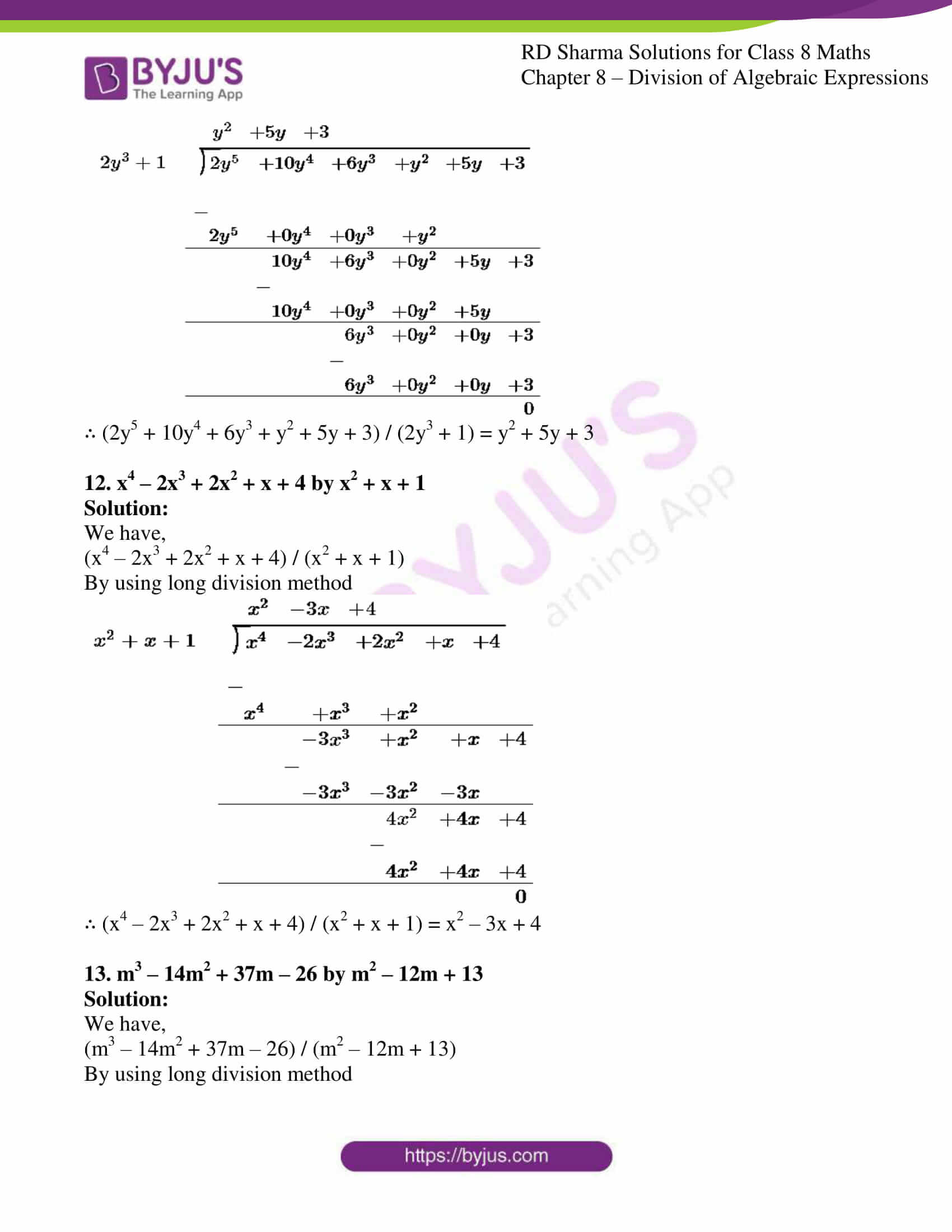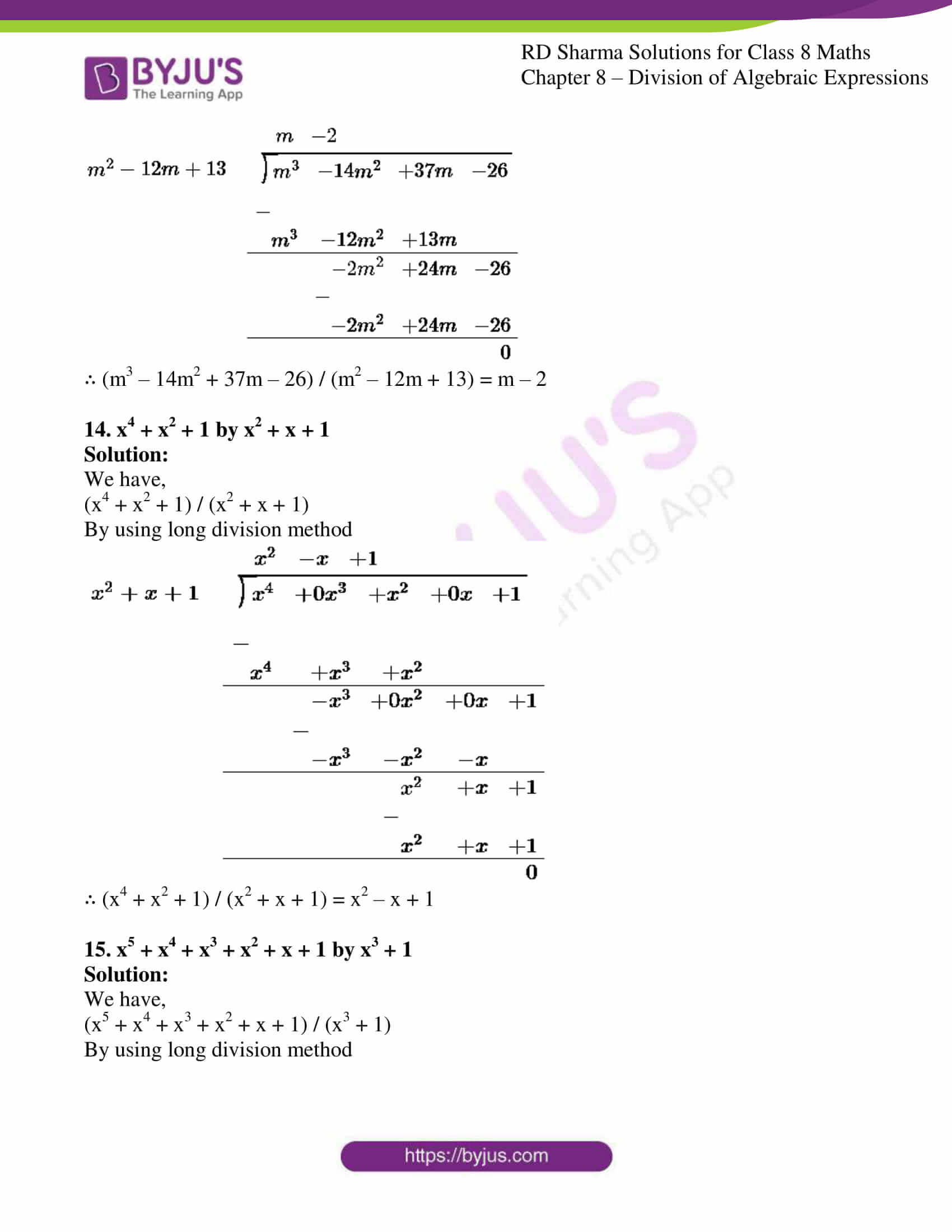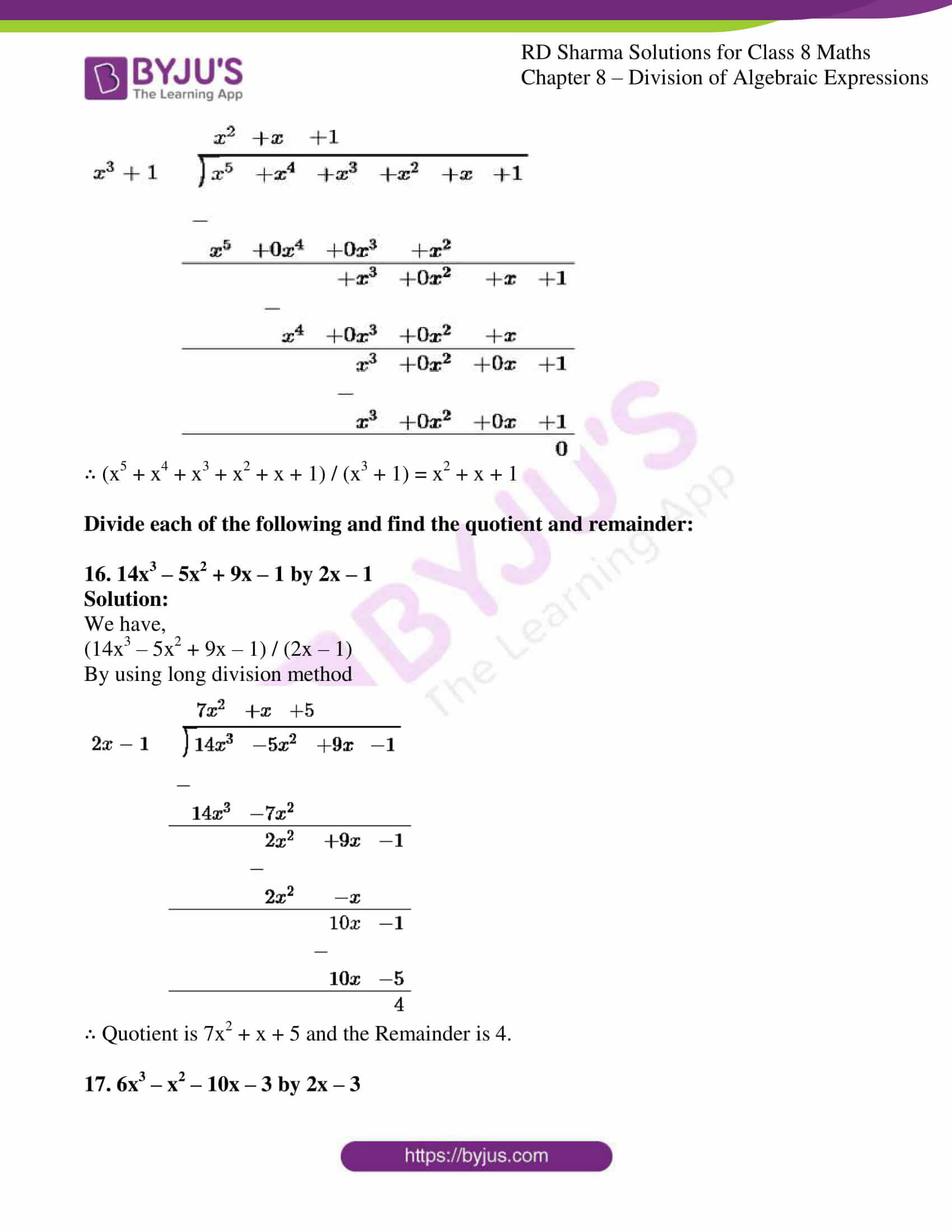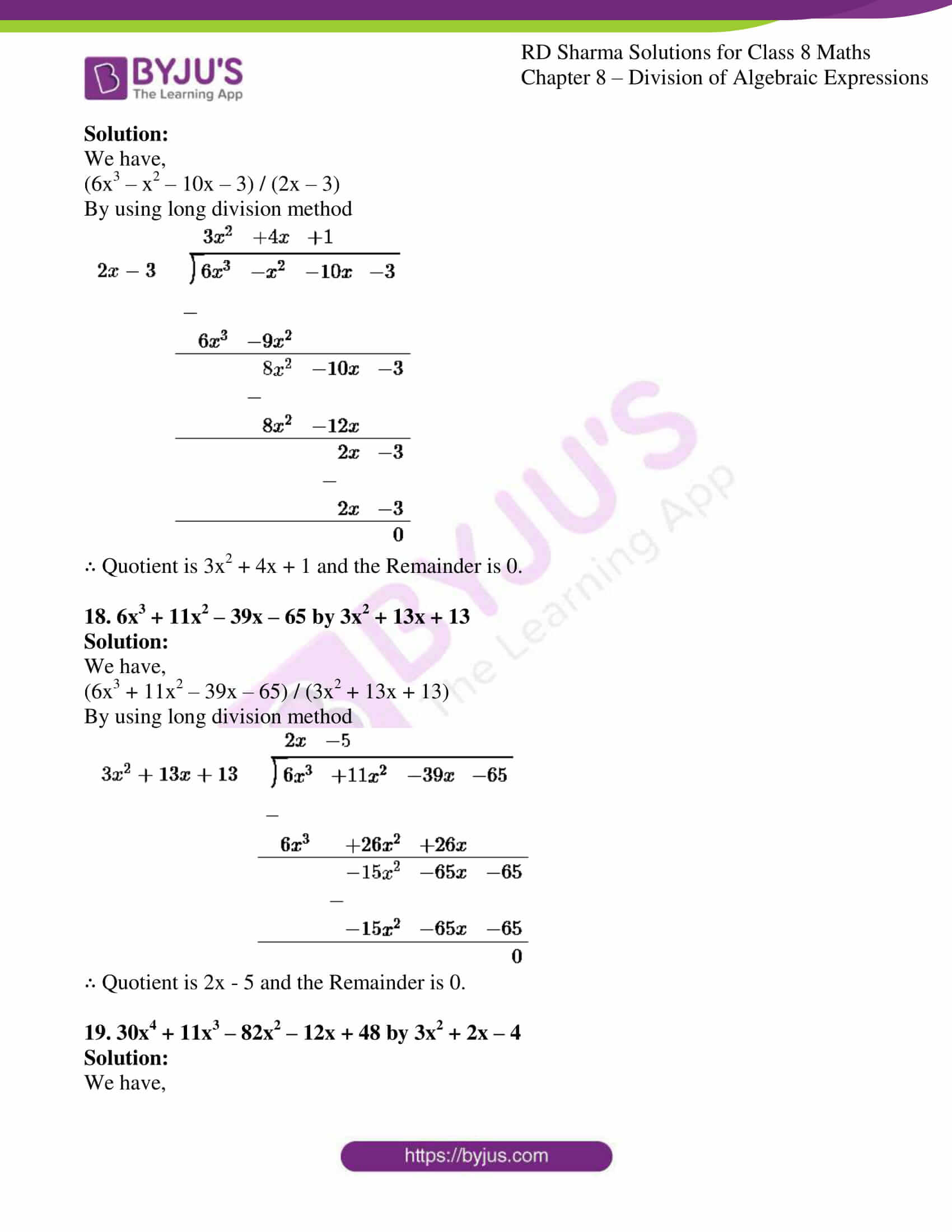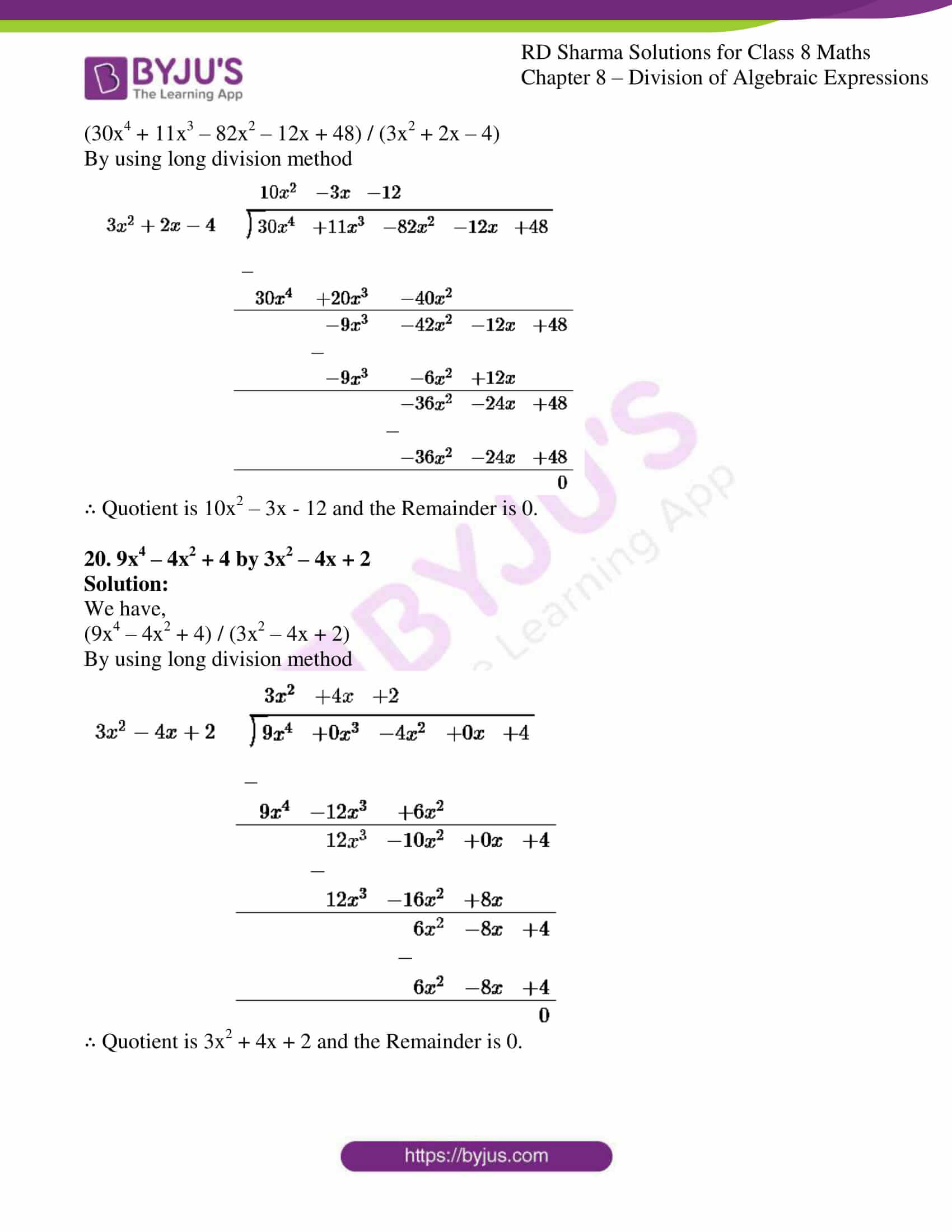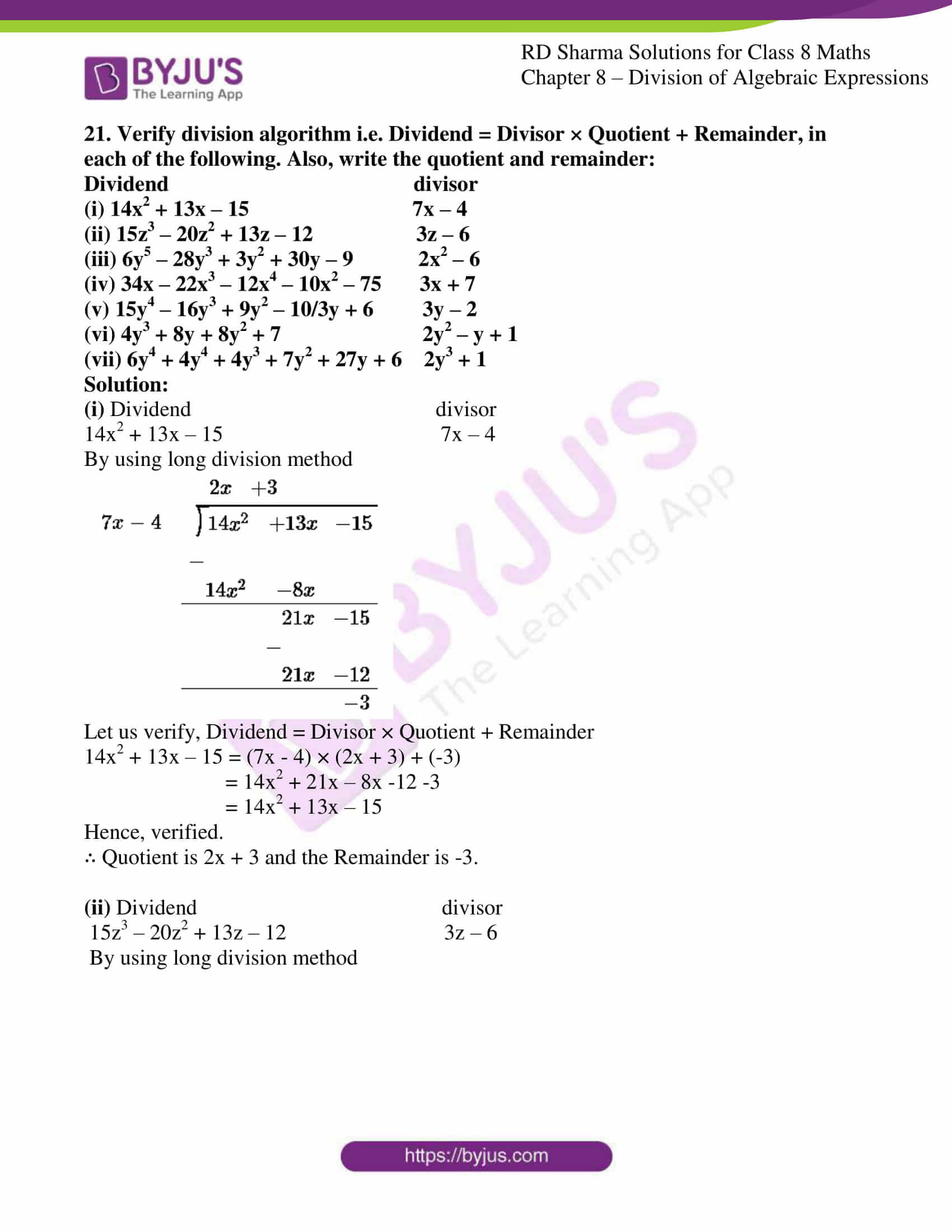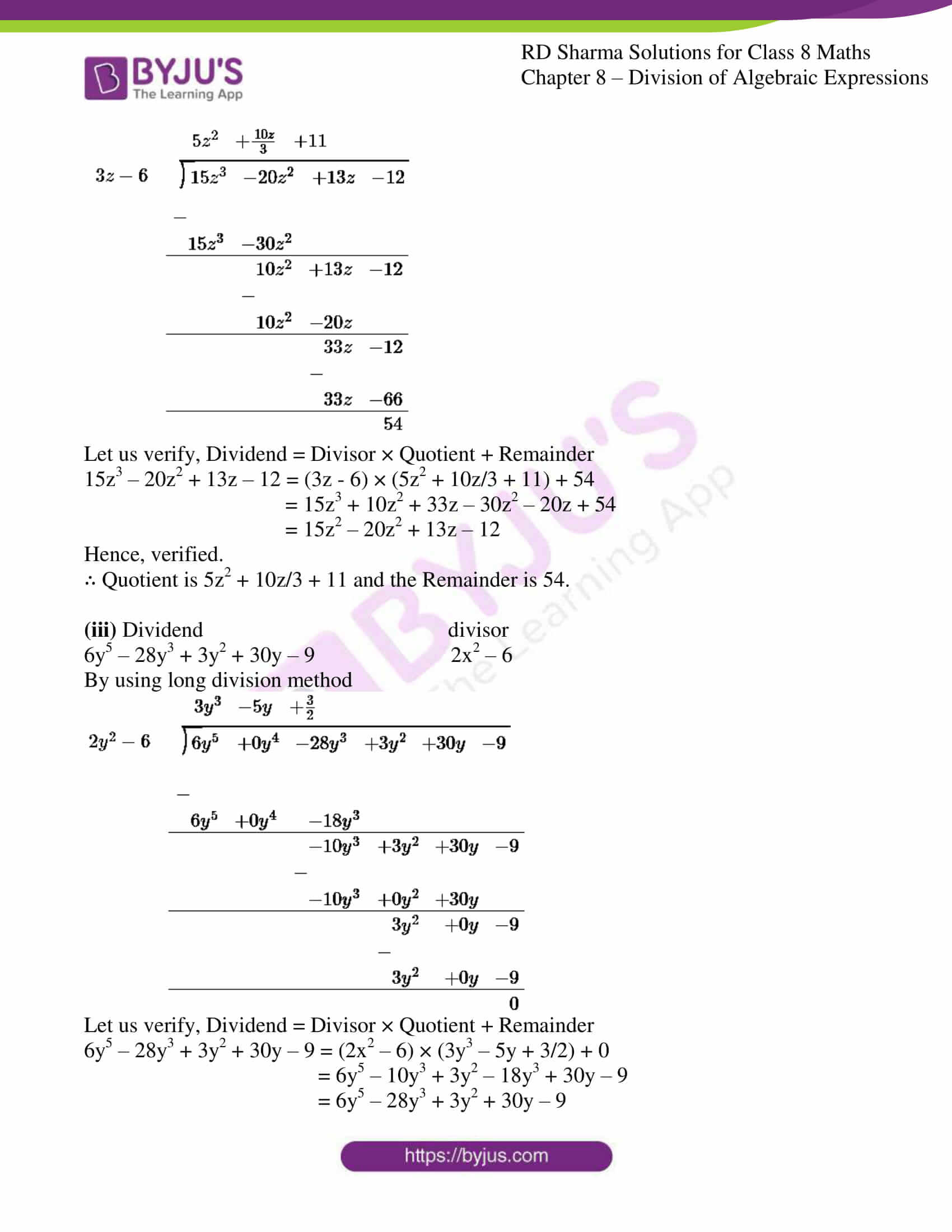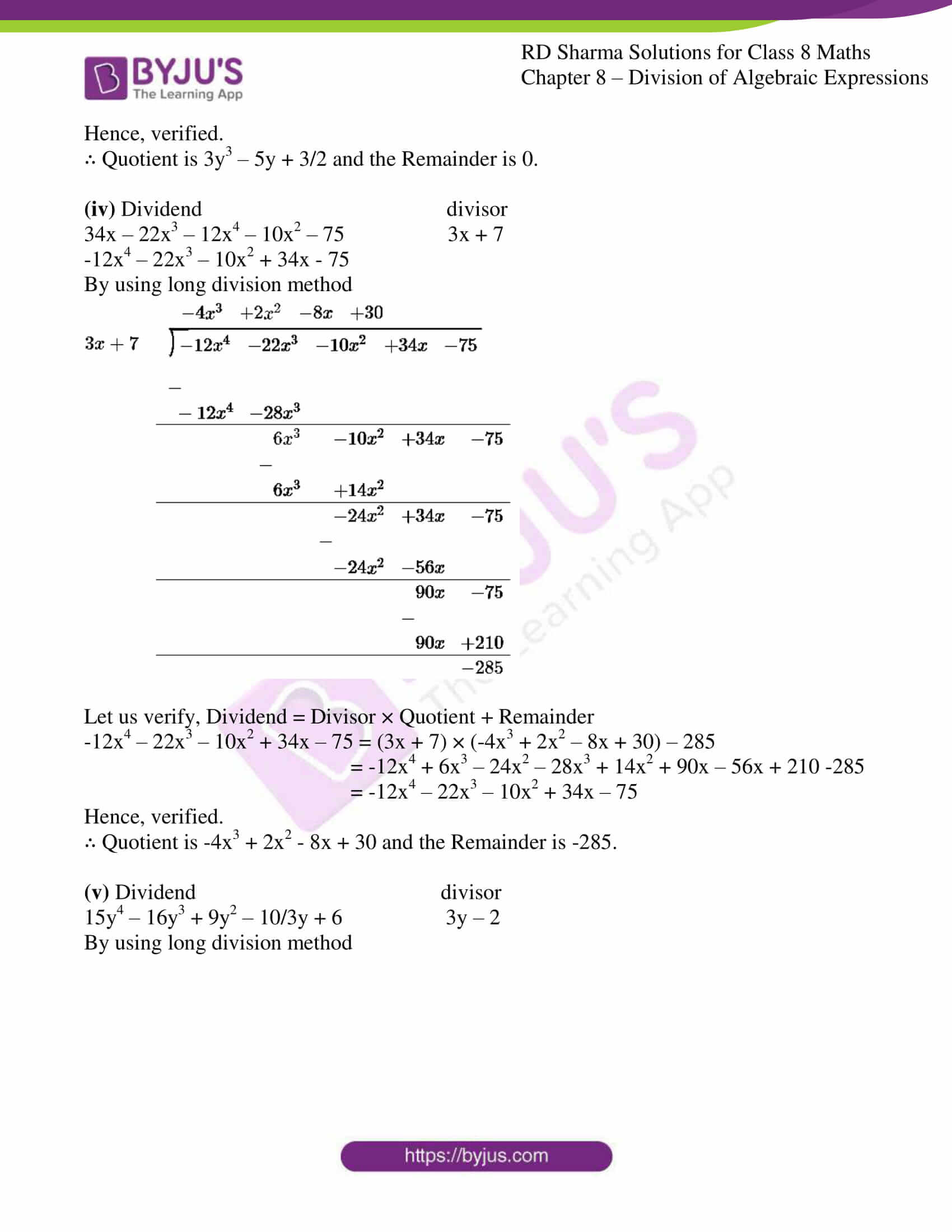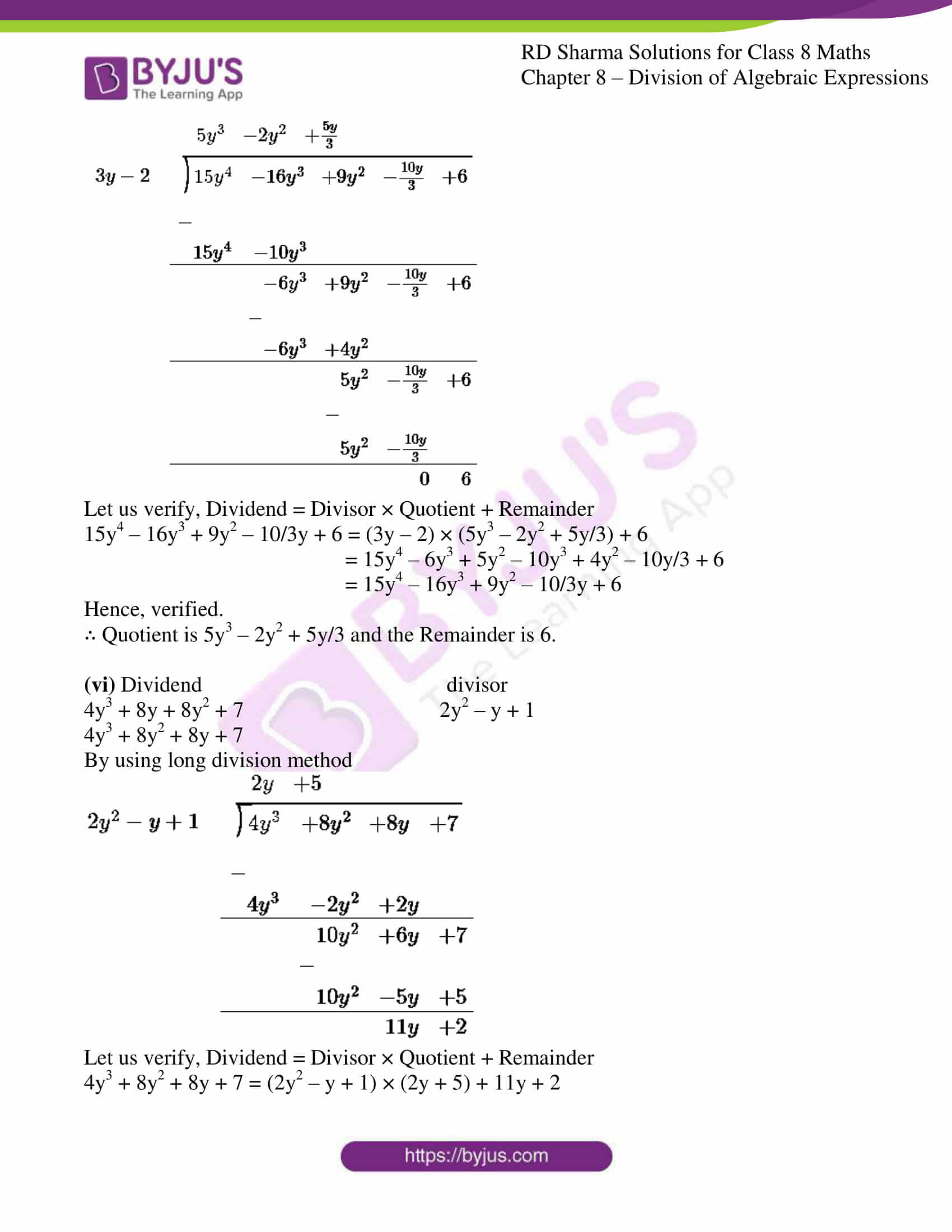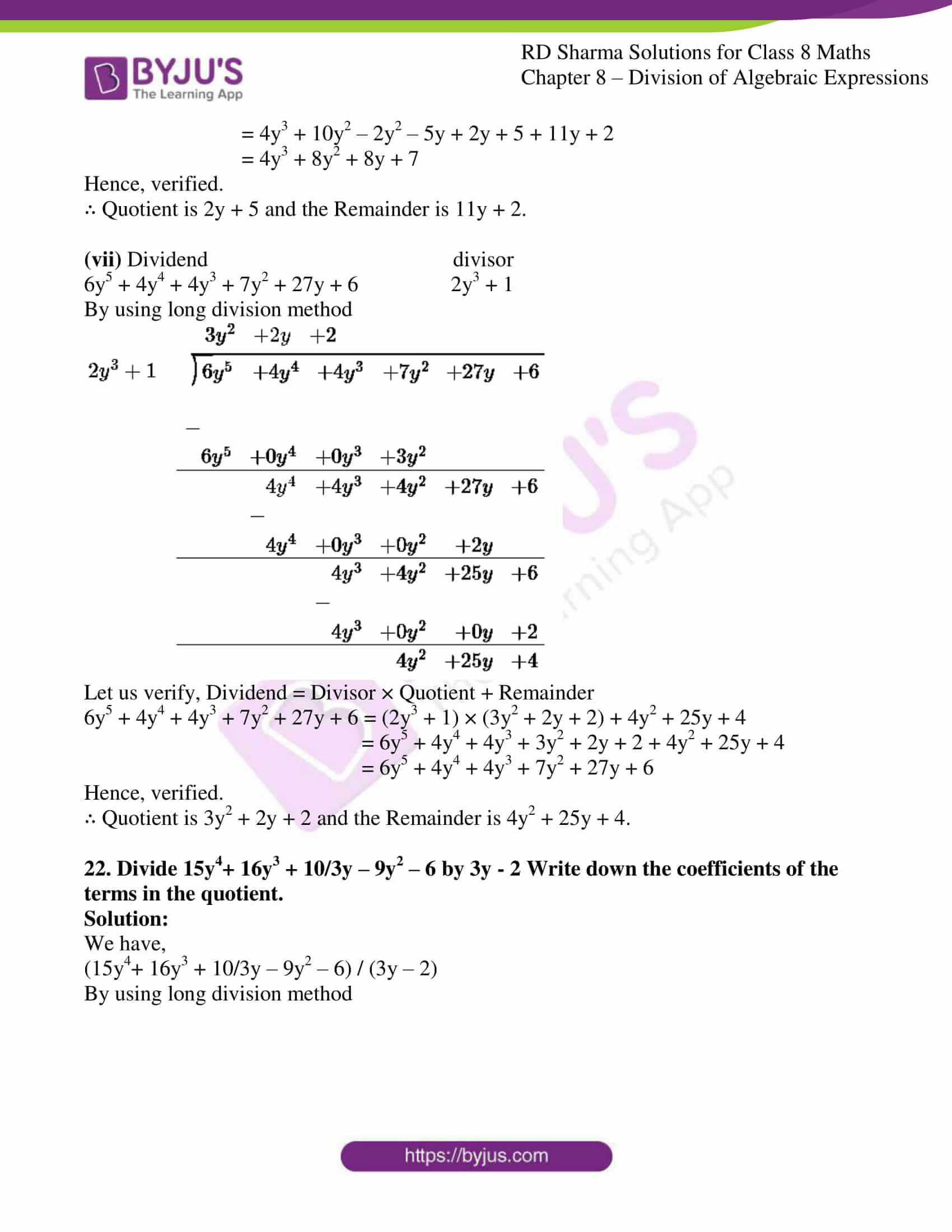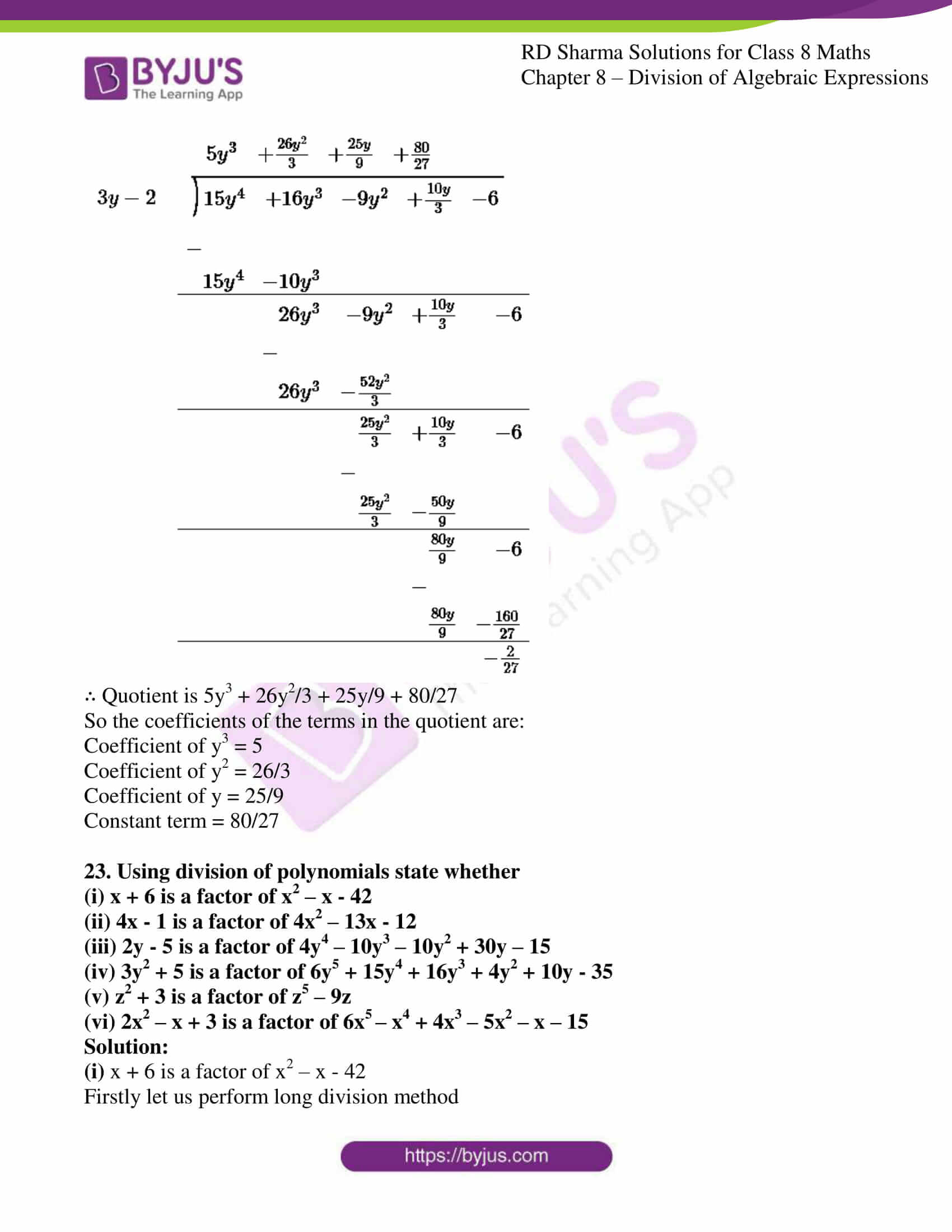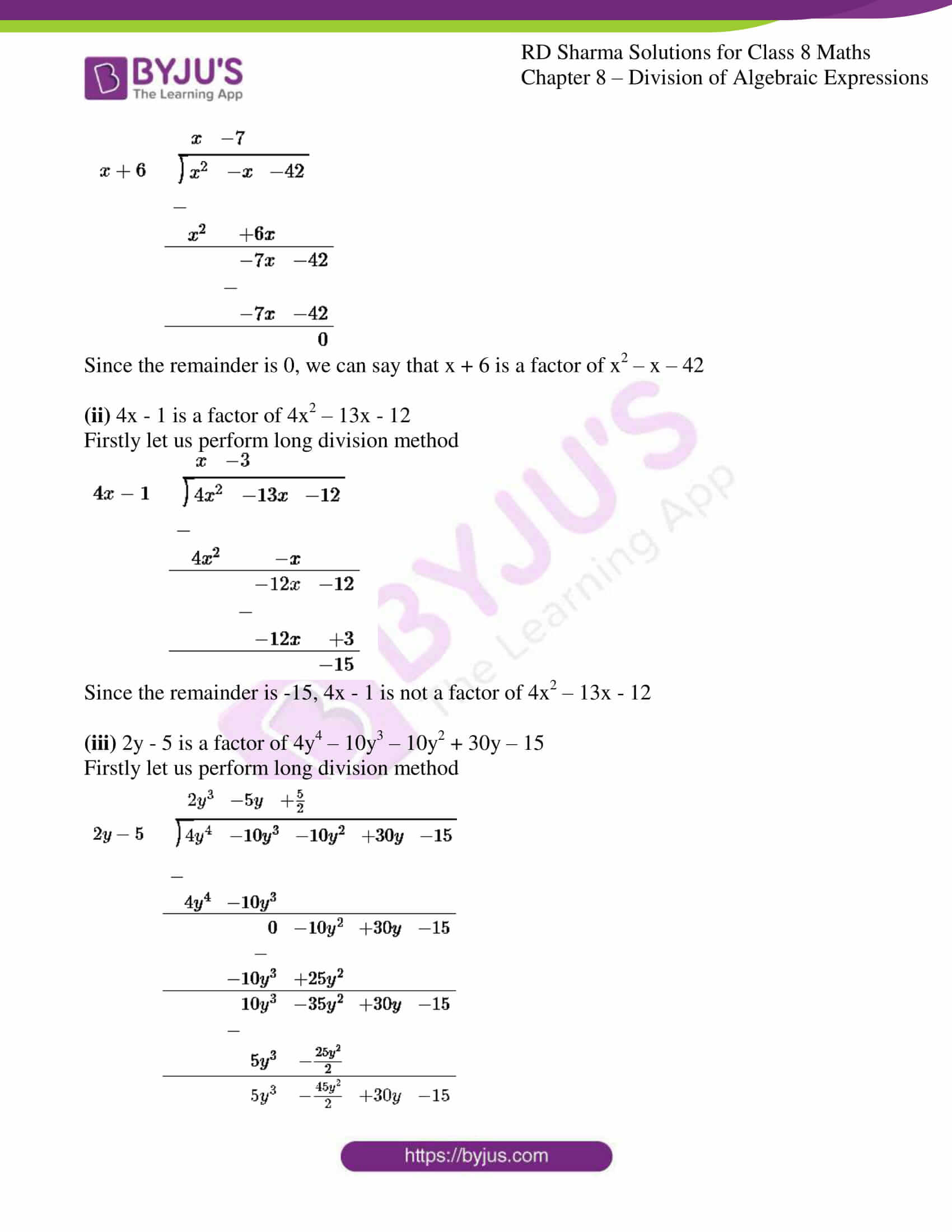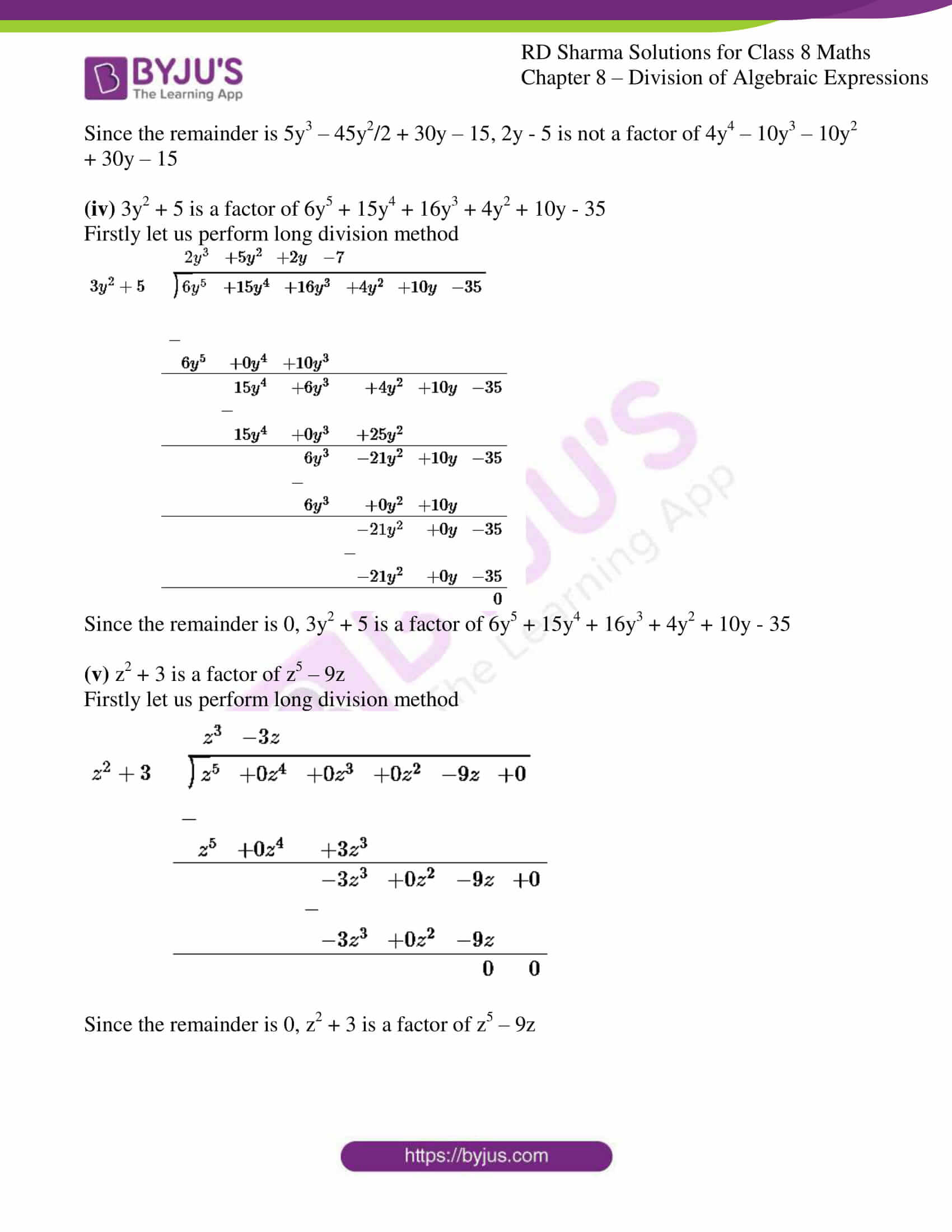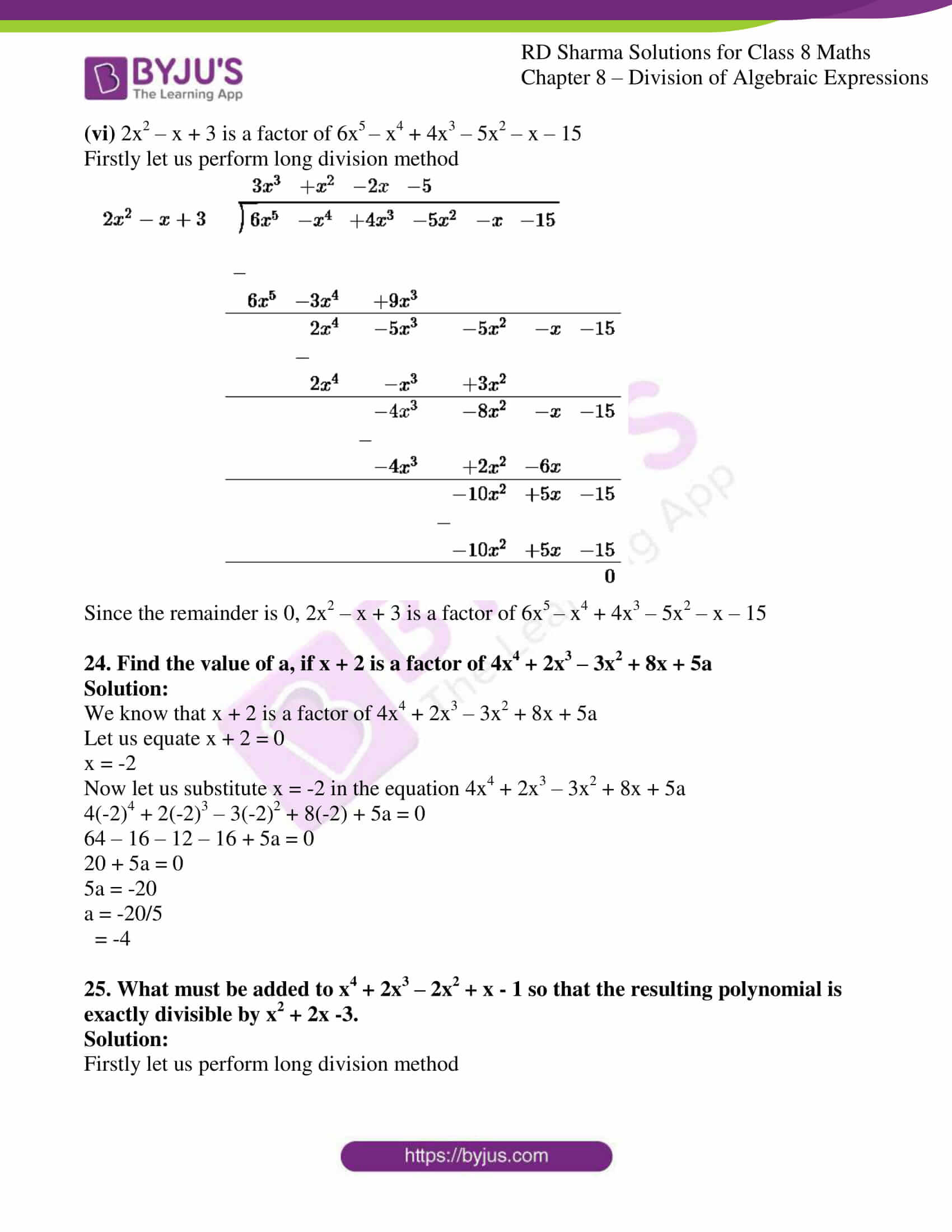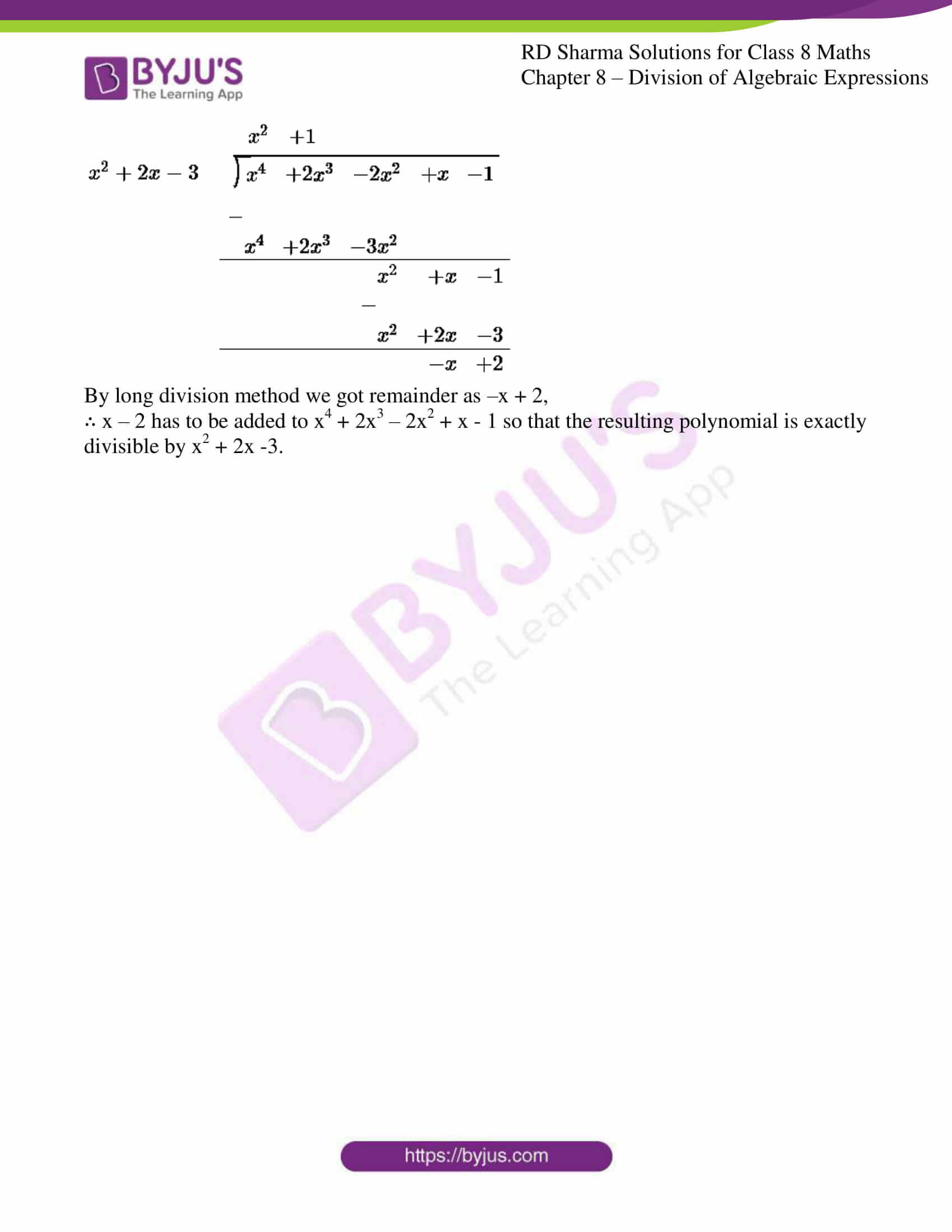### Access Answers to RD Sharma Solutions for Class 8 Maths Exercise 8.4 Chapter 8 Division of Algebraic Expressions

#### EXERCISE 8.4 PAGE NO: 8.11

Divide:

1. 5x3 – 15x2 + 25x by 5x

Solution:

We have,

(5x3 – 15x2 + 25x) / 5x

5x3/5x – 15x2/5x + 25x/5x

By using the formula an / am = an-m

5/5 x3-1 – 15/5 x2-1 + 25/5 x1-1

x2 – 3x + 5

2. 4z3 + 6z2 – z by -1/2z

Solution:

We have,

(4z3 + 6z2 – z) / -1/2z

4z3/(-1/2z) + 6z2/(-1/2z) – z/(-1/2z)

By using the formula an / am = an-m

-8 z3-1 – 12z2-1 + 2 z1-1

-8z2 – 12z + 2

3. 9x2y – 6xy + 12xy2 by -3/2xy

Solution:

We have,

(9x2y – 6xy + 12xy2) / -3/2xy

9x2y/(-3/2xy) – 6xy/(-3/2xy) + 12xy2/(-3/2xy)

By using the formula an / am = an-m

(-9×2)/3 x2-1y1-1 – (-6×2)/3 x1-1y1-1 + (-12×2)/3 x1-1y2-1

-6x + 4 – 8y

4. 3x3y2 + 2x2y + 15xy by 3xy

Solution:

We have,

(3x3y2 + 2x2y + 15xy) / 3xy

3x3y2/3xy + 2x2y/3xy + 15xy/3xy

By using the formula an / am = an-m

3/3 x3-1y2-1 + 2/3 x2-1y1-1 + 15/3 x1-1y1-1

x2y + 2/3x + 5

5. x2 + 7x + 12 by x + 4

Solution:

We have,

(x2 + 7x + 12) / (x + 4)

By using long division method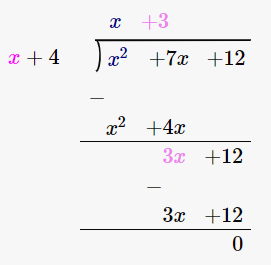∴ (x2 + 7x + 12) / (x + 4) = x + 3

6. 4y2 + 3y + 1/2 by 2y + 1

Solution:

We have,

4y2 + 3y + 1/2 by (2y + 1)

By using long division method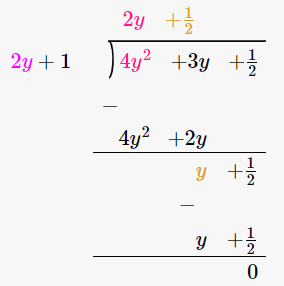∴ (4y2 + 3y + 1/2) / (2y + 1) = 2y + 1/2

7. 3x3 + 4x2 + 5x + 18 by x + 2

Solution:

We have,

(3x3 + 4x2 + 5x + 18) / (x + 2)

By using long division method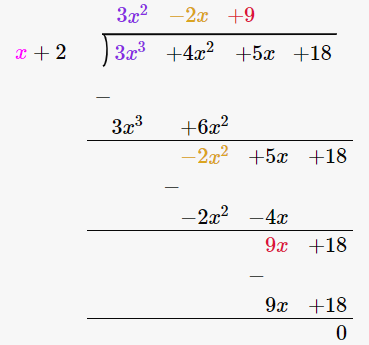∴ (3x3 + 4x2 + 5x + 18) / (x + 2) = 3x2 – 2x + 9

8. 14x2 – 53x + 45 by 7x – 9

Solution:

We have,

(14x2 – 53x + 45) / (7x – 9)

By using long division method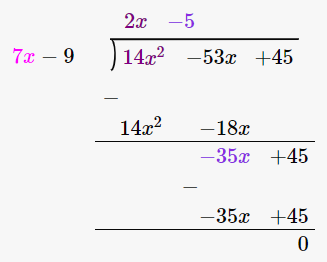∴ (14x2 – 53x + 45) / (7x – 9) = 2x – 5

9. -21 + 71x – 31x2 – 24x3 by 3 – 8x

Solution:

We have,

-21 + 71x – 31x2 – 24x3 by 3 – 8x

(-24x3 – 31x2 + 71x – 21) / (3 – 8x)

By using long division method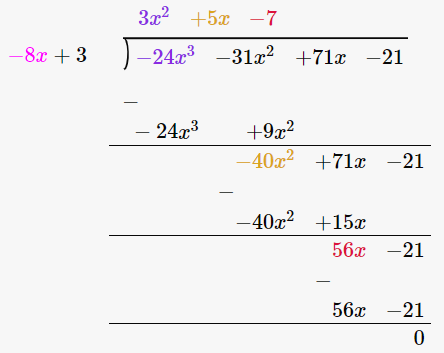∴ (-24x3 – 31x2 + 71x – 21) / (3 – 8x) = 3x2 + 5x – 7

10. 3y4 – 3y3 – 4y2 – 4y by y2 – 2y

Solution:

We have,

(3y4 – 3y3 – 4y2 – 4y) / (y2 – 2y)

By using long division method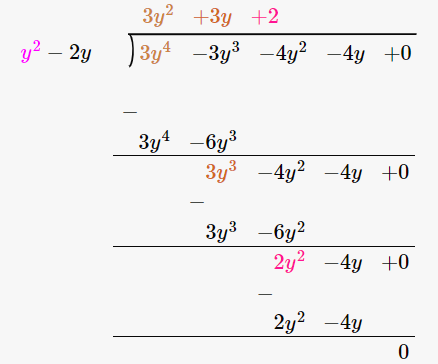∴ (3y4 – 3y3 – 4y2 – 4y) / (y2 – 2y) = 3y2 + 3y + 2

11. 2y5 + 10y4 + 6y3 + y2 + 5y + 3 by 2y3 + 1

Solution:

We have,

(2y5 + 10y4 + 6y3 + y2 + 5y + 3) / (2y3 + 1)

By using long division method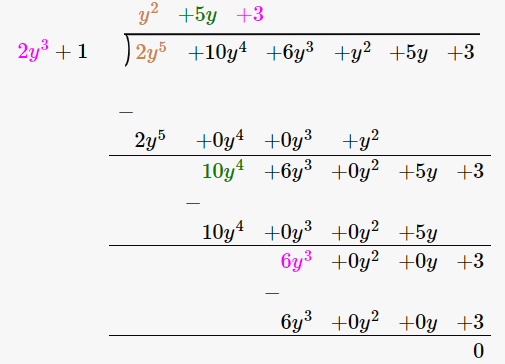∴ (2y5 + 10y4 + 6y3 + y2 + 5y + 3) / (2y3 + 1) = y2 + 5y + 3

12. x4 – 2x3 + 2x2 + x + 4 by x2 + x + 1

Solution:

We have,

(x4 – 2x3 + 2x2 + x + 4) / (x2 + x + 1)

By using long division method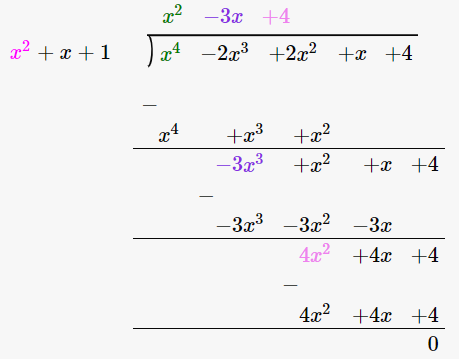∴ (x4 – 2x3 + 2x2 + x + 4) / (x2 + x + 1) = x2 – 3x + 4

13. m3 – 14m2 + 37m – 26 by m2 – 12m + 13

Solution:

We have,

(m3 – 14m2 + 37m – 26) / (m2 – 12m + 13)

By using long division method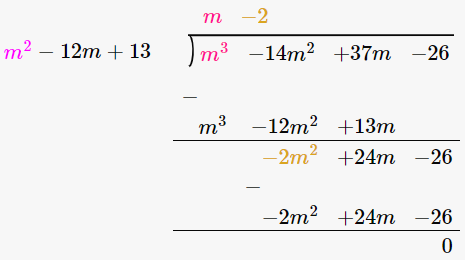∴ (m3 – 14m2 + 37m – 26) / (m2 – 12m + 13) = m – 2

14. x4 + x2 + 1 by x2 + x + 1

Solution:

We have,

(x4 + x2 + 1) / (x2 + x + 1)

By using long division method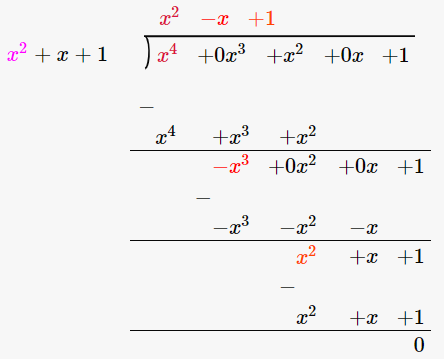∴ (x4 + x2 + 1) / (x2 + x + 1) = x2 – x + 1

15. x5 + x4 + x3 + x2 + x + 1 by x3 + 1

Solution:

We have,

(x5 + x4 + x3 + x2 + x + 1) / (x3 + 1)

By using long division method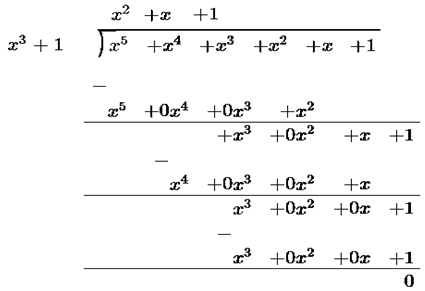∴ (x5 + x4 + x3 + x2 + x + 1) / (x3 + 1) = x2 + x + 1

Divide each of the following and find the quotient and remainder:

16. 14x3 – 5x2 + 9x – 1 by 2x – 1

Solution:

We have,

(14x3 – 5x2 + 9x – 1) / (2x – 1)

By using long division method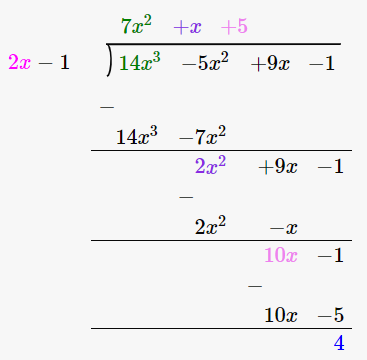∴ Quotient is 7x2 + x + 5 and the Remainder is 4.

17. 6x3 – x2 – 10x – 3 by 2x – 3

Solution:

We have,

(6x3 – x2 – 10x – 3) / (2x – 3)

By using long division method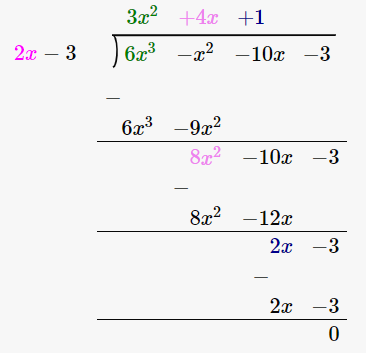∴ Quotient is 3x2 + 4x + 1 and the Remainder is 0.

18. 6x3 + 11x2 – 39x – 65 by 3x2 + 13x + 13

Solution:

We have,

(6x3 + 11x2 – 39x – 65) / (3x2 + 13x + 13)

By using long division method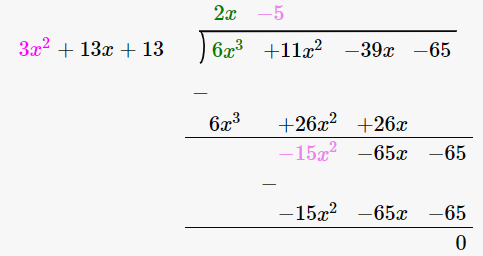∴ Quotient is 2x – 5 and the Remainder is 0.

19. 30x4 + 11x3 – 82x2 – 12x + 48 by 3x2 + 2x – 4

Solution:

We have,

(30x4 + 11x3 – 82x2 – 12x + 48) / (3x2 + 2x – 4)

By using long division method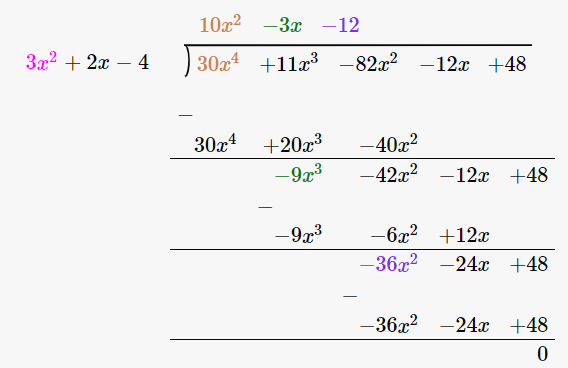∴ Quotient is 10x2 – 3x – 12 and the Remainder is 0.

20. 9x4 – 4x2 + 4 by 3x2 – 4x + 2

Solution:

We have,

(9x4 – 4x2 + 4) / (3x2 – 4x + 2)

By using long division method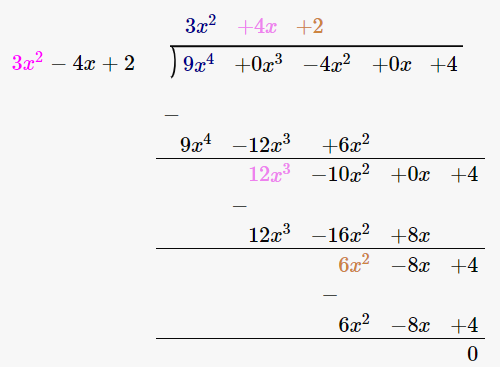∴ Quotient is 3x2 + 4x + 2 and the Remainder is 0.

21. Verify division algorithm i.e. Dividend = Divisor × Quotient + Remainder, in each of the following. Also, write the quotient and remainder:

Dividend divisor

(i) 14x2 + 13x – 15 7x – 4

(ii) 15z3 – 20z2 + 13z – 12 3z – 6

(iii) 6y5 – 28y3 + 3y2 + 30y – 9 2x2 – 6

(iv) 34x – 22x3 – 12x4 – 10x2 – 75 3x + 7

(v) 15y4 – 16y3 + 9y2 – 10/3y + 6 3y – 2

(vi) 4y3 + 8y + 8y2 + 7 2y2 – y + 1

(vii) 6y4 + 4y4 + 4y3 + 7y2 + 27y + 6 2y3 + 1

Solution:

(i) Dividend divisor

14x2 + 13x – 15 7x – 4

By using long division method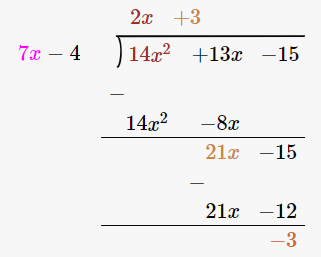Let us verify, Dividend = Divisor × Quotient + Remainder

14x2 + 13x – 15 = (7x – 4) × (2x + 3) + (-3)

= 14x2 + 21x – 8x -12 -3

= 14x2 + 13x – 15

Hence, verified.

∴ Quotient is 2x + 3 and the Remainder is -3.

(ii) Dividend divisor

15z3 – 20z2 + 13z – 12 3z – 6

By using long division method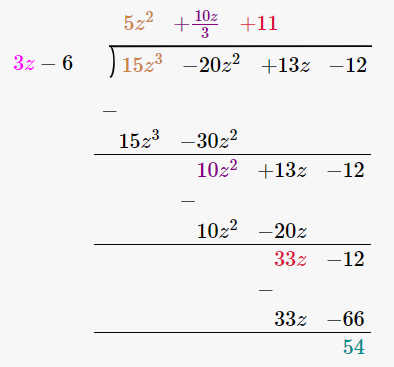Let us verify, Dividend = Divisor × Quotient + Remainder

15z3 – 20z2 + 13z – 12 = (3z – 6) × (5z2 + 10z/3 + 11) + 54

= 15z3 + 10z2 + 33z – 30z2 – 20z + 54

= 15z2 – 20z2 + 13z – 12

Hence, verified.

∴ Quotient is 5z2 + 10z/3 + 11 and the Remainder is 54.

(iii) Dividend divisor

6y5 – 28y3 + 3y2 + 30y – 9 2x2 – 6

By using long division method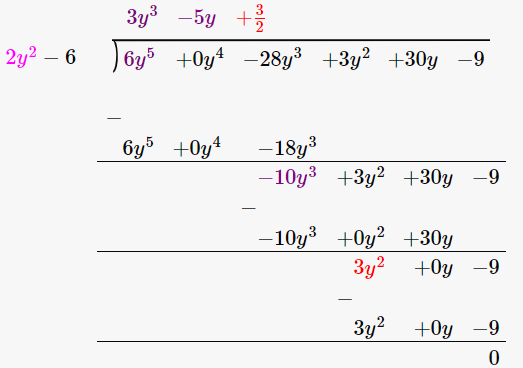Let us verify, Dividend = Divisor × Quotient + Remainder

6y5 – 28y3 + 3y2 + 30y – 9 = (2x2 – 6) × (3y3 – 5y + 3/2) + 0

= 6y5 – 10y3 + 3y2 – 18y3 + 30y – 9

= 6y5 – 28y3 + 3y2 + 30y – 9

Hence, verified.

∴ Quotient is 3y3 – 5y + 3/2 and the Remainder is 0.

(iv) Dividend divisor

34x – 22x3 – 12x4 – 10x2 – 75 3x + 7

-12x4 – 22x3 – 10x2 + 34x – 75

By using long division method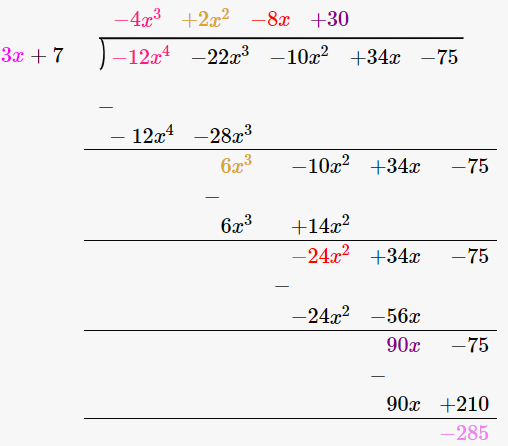Let us verify, Dividend = Divisor × Quotient + Remainder

-12x4 – 22x3 – 10x2 + 34x – 75 = (3x + 7) × (-4x3 + 2x2 – 8x + 30) – 285

= -12x4 + 6x3 – 24x2 – 28x3 + 14x2 + 90x – 56x + 210 -285

= -12x4 – 22x3 – 10x2 + 34x – 75

Hence, verified.

∴ Quotient is -4x3 + 2x2 – 8x + 30 and the Remainder is -285.

(v) Dividend divisor

15y4 – 16y3 + 9y2 – 10/3y + 6 3y – 2

By using long division method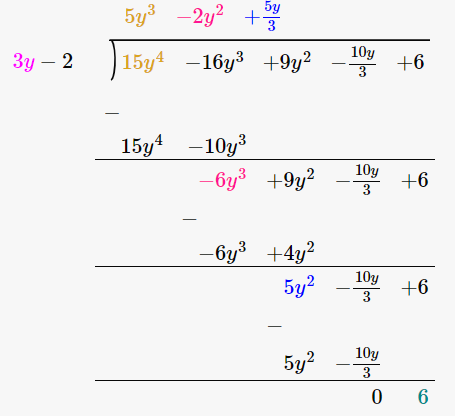Let us verify, Dividend = Divisor × Quotient + Remainder

15y4 – 16y3 + 9y2 – 10/3y + 6 = (3y – 2) × (5y3 – 2y2 + 5y/3) + 6

= 15y4 – 6y3 + 5y2 – 10y3 + 4y2 – 10y/3 + 6

= 15y4 – 16y3 + 9y2 – 10/3y + 6

Hence, verified.

∴ Quotient is 5y3 – 2y2 + 5y/3 and the Remainder is 6.

(vi) Dividend divisor

4y3 + 8y + 8y2 + 7 2y2 – y + 1

4y3 + 8y2 + 8y + 7

By using long division method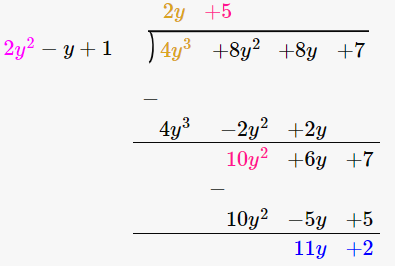Let us verify, Dividend = Divisor × Quotient + Remainder

4y3 + 8y2 + 8y + 7 = (2y2 – y + 1) × (2y + 5) + 11y + 2

= 4y3 + 10y2 – 2y2 – 5y + 2y + 5 + 11y + 2

= 4y3 + 8y2 + 8y + 7

Hence, verified.

∴ Quotient is 2y + 5 and the Remainder is 11y + 2.

(vii) Dividend divisor

6y5 + 4y4 + 4y3 + 7y2 + 27y + 6 2y3 + 1

By using long division method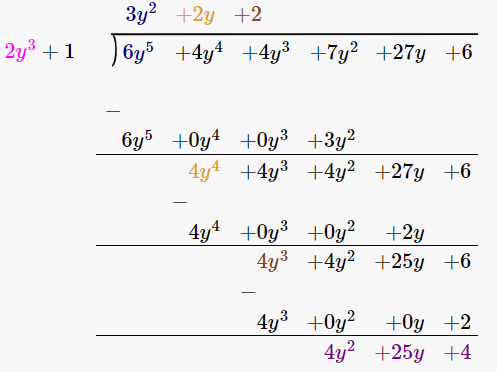Let us verify, Dividend = Divisor × Quotient + Remainder

6y5 + 4y4 + 4y3 + 7y2 + 27y + 6 = (2y3 + 1) × (3y2 + 2y + 2) + 4y2 + 25y + 4

= 6y5 + 4y4 + 4y3 + 3y2 + 2y + 2 + 4y2 + 25y + 4

= 6y5 + 4y4 + 4y3 + 7y2 + 27y + 6

Hence, verified.

∴ Quotient is 3y2 + 2y + 2 and the Remainder is 4y2 + 25y + 4.

22. Divide 15y4+ 16y3 + 10/3y – 9y2 – 6 by 3y – 2 Write down the coefficients of the terms in the quotient.

Solution:

We have,

(15y4+ 16y3 + 10/3y – 9y2 – 6) / (3y – 2)

By using long division method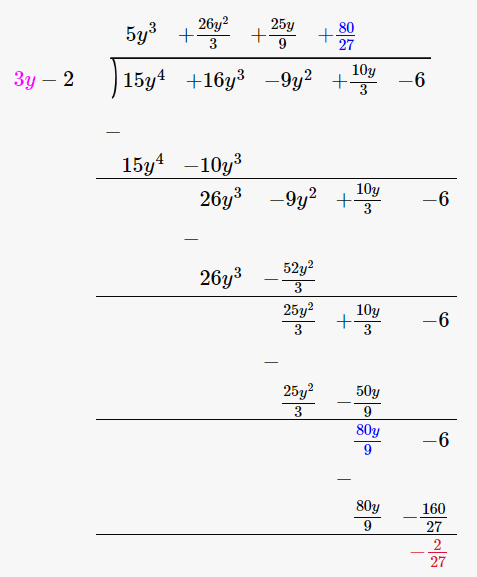∴ Quotient is 5y3 + 26y2/3 + 25y/9 + 80/27

So the coefficients of the terms in the quotient are:

Coefficient of y3 = 5

Coefficient of y2 = 26/3

Coefficient of y = 25/9

Constant term = 80/27

23. Using division of polynomials state whether
(i) x + 6 is a factor of x2 – x – 42
(ii) 4x – 1 is a factor of 4x2 – 13x – 12
(iii) 2y – 5 is a factor of 4y4 – 10y3 – 10y2 + 30y – 15
(iv) 3y2 + 5 is a factor of 6y5 + 15y4 + 16y3 + 4y2 + 10y – 35
(v) z2 + 3 is a factor of z5 – 9z
(vi) 2x2 – x + 3 is a factor of 6x5 – x4 + 4x3 – 5x2 – x – 15

Solution:

(i) x + 6 is a factor of x2 – x – 42

Firstly let us perform long division method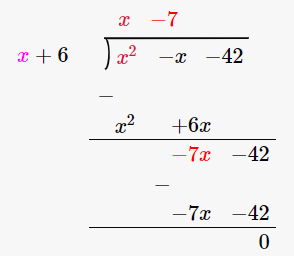Since the remainder is 0, we can say that x + 6 is a factor of x2 – x – 42

(ii) 4x – 1 is a factor of 4x2 – 13x – 12

Firstly let us perform long division method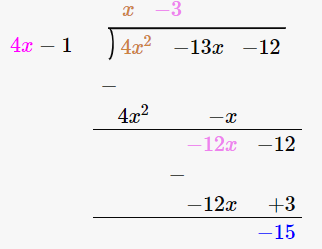Since the remainder is -15, 4x – 1 is not a factor of 4x2 – 13x – 12

(iii) 2y – 5 is a factor of 4y4 – 10y3 – 10y2 + 30y – 15

Firstly let us perform long division method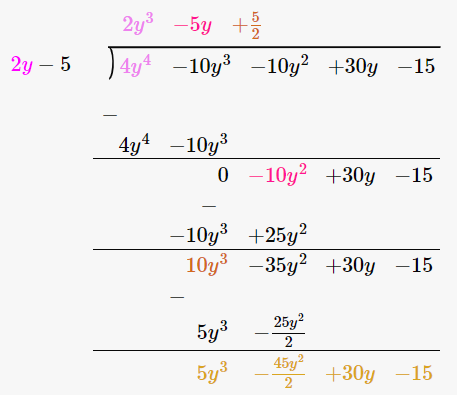Since the remainder is 5y3 – 45y2/2 + 30y – 15, 2y – 5 is not a factor of 4y4 – 10y3 – 10y2 + 30y – 15

(iv) 3y2 + 5 is a factor of 6y5 + 15y4 + 16y3 + 4y2 + 10y – 35

Firstly let us perform long division method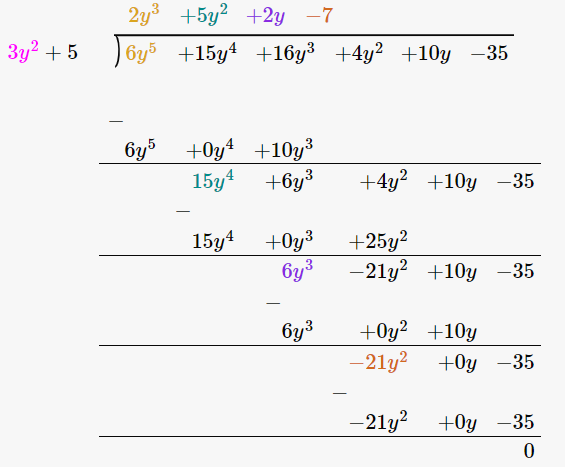Since the remainder is 0, 3y2 + 5 is a factor of 6y5 + 15y4 + 16y3 + 4y2 + 10y – 35

(v) z2 + 3 is a factor of z5 – 9z

Firstly let us perform long division method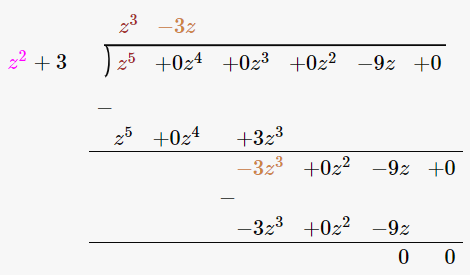Since the remainder is 0, z2 + 3 is a factor of z5 – 9z

(vi) 2x2 – x + 3 is a factor of 6x5 – x4 + 4x3 – 5x2 – x – 15

Firstly let us perform long division method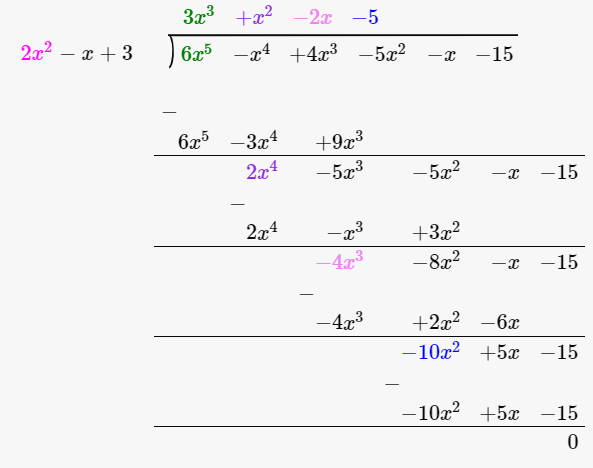Since the remainder is 0, 2x2 – x + 3 is a factor of 6x5 – x4 + 4x3 – 5x2 – x – 15

24. Find the value of a, if x + 2 is a factor of 4x4 + 2x3 – 3x2 + 8x + 5a

Solution:

We know that x + 2 is a factor of 4x4 + 2x3 – 3x2 + 8x + 5a

Let us equate x + 2 = 0

x = -2

Now let us substitute x = -2 in the equation 4x4 + 2x3 – 3x2 + 8x + 5a

4(-2)4 + 2(-2)3 – 3(-2)2 + 8(-2) + 5a = 0

64 – 16 – 12 – 16 + 5a = 0

20 + 5a = 0

5a = -20

a = -20/5

= -4

25. What must be added to x4 + 2x3 – 2x2 + x – 1 so that the resulting polynomial is exactly divisible by x2 + 2x -3.

Solution:

Firstly let us perform long division method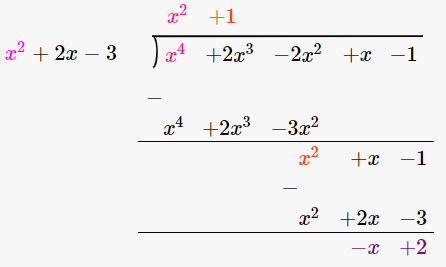By long division method we got remainder as –x + 2,

∴ x – 2 has to be added to x4 + 2x3 – 2x2 + x – 1 so that the resulting polynomial is exactly divisible by x2 + 2x -3.